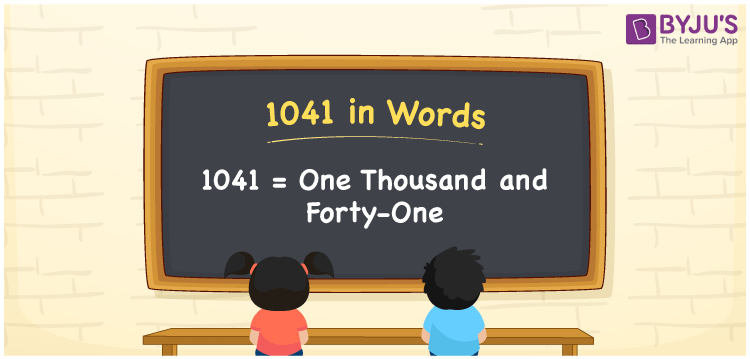# 1041 in Words

We can write 1041 in words as One thousand and forty-one. If you spent Rs. 1041 for designing a study table, you can say, “I spent One thousand and forty-one rupees to design or makeover the study table”. Also, it is important to recall that 1041 is a cardinal number since it conveys a specific quantity or amount.

 1041 in words One thousand and forty-one One thousand and forty-one in Numbers 1041

## 1041 in English Words

We generally express numbers in words using the English alphabet. So, we can spell 1041 in English as “One thousand and forty-one”.## How to Write 1041 in Words?

The conversion of the numeral 1041 to words is effortless when we use the place value chart. The below table shows the place values for all digits of the number 1041.

 Thousands Hundreds Tens Ones 1 0 4 1

Here, ones = 1, tens = 4, hundreds = 0, thousands = 1

By expanding these numbers, we get;

1 × Thousand + 0 × Hundred + 4 × Ten + 1 × One

= 1 × 1000 + 0 × 100 + 4 × 10 + 1 × 1

= 1000 + 40 + 1

= 1000 + 41

= One thousand + Forty-one

= One thousand and forty-one

Therefore, 1041 in words = One thousand and forty-one.

As we know, 1041 is a natural number that precedes 1042 and succeeds 1040.

1041 in words – One thousand and forty-one

Is 1041 an even number? – No

Is 1041 an odd number? – Yes

Is 1041 a perfect square number? – No

Is 1041 a perfect cube number? – No

Is 1041 a prime number? – No

Is 1041 a composite number? – Yes

## Frequently Asked Questions on 1041 in Words

Q1

### How do you write 1041 in words?

We can spell the number 1041 in English words as One thousand and forty-one.
Q2

### Express the value of 1041 – 335 in words.

1041 – 335 = 706 So, the value of 1041 – 335, i.e., 706 is expressed in words as Seven hundred and six.
Q3

### How to write Rs. 1041 in words on a cheque?

On a cheque, Rs. 1041 can be written in words as “One thousand and forty-one rupees only”.